Select Page

Deep Learning and Machine Learning are no longer a novelty. Many applications are utilizing the power of these technologies for cheap predictions, object detection and various other purposes. In this article, we cover the Linear Regression. You will learn how Linear Regression functions, what is Multiple Linear Regression, implement both algorithms from scratch and with ML.NET. Linear Regression is a well-known algorithm and it is the basics of this vast field. In a way, it is the root of it all.

Are you afraid that AI might take your job? Make sure you are the one who is building it.

STAY RELEVANT IN THE RISING AI INDUSTRY! 🖖

## 1. Prerequisites and Dataset

What we want to do in this article, is to make an algorithm that is able to predict the price of the house based on the provided parameters. This algorithm should learn how to do that using the famous Boston Housing Dataset. This dataset is composed of 12 features and contains information collected by the U.S Census Service concerning housing in the area of Boston Mass. It is a small dataset with only 506 samples.The implementations provided here are done in C#, and we use the latest .NET 5. So make sure that you have installed this SDK. If you are using Visual Studio this comes with version 16.8.3. Also, make sure that you have installed the following packages:

``````Install-Package Microsoft.ML
Install-Package MathNet``````

You can do a similar thing using Visual Studio’s Manage NuGetPackage option: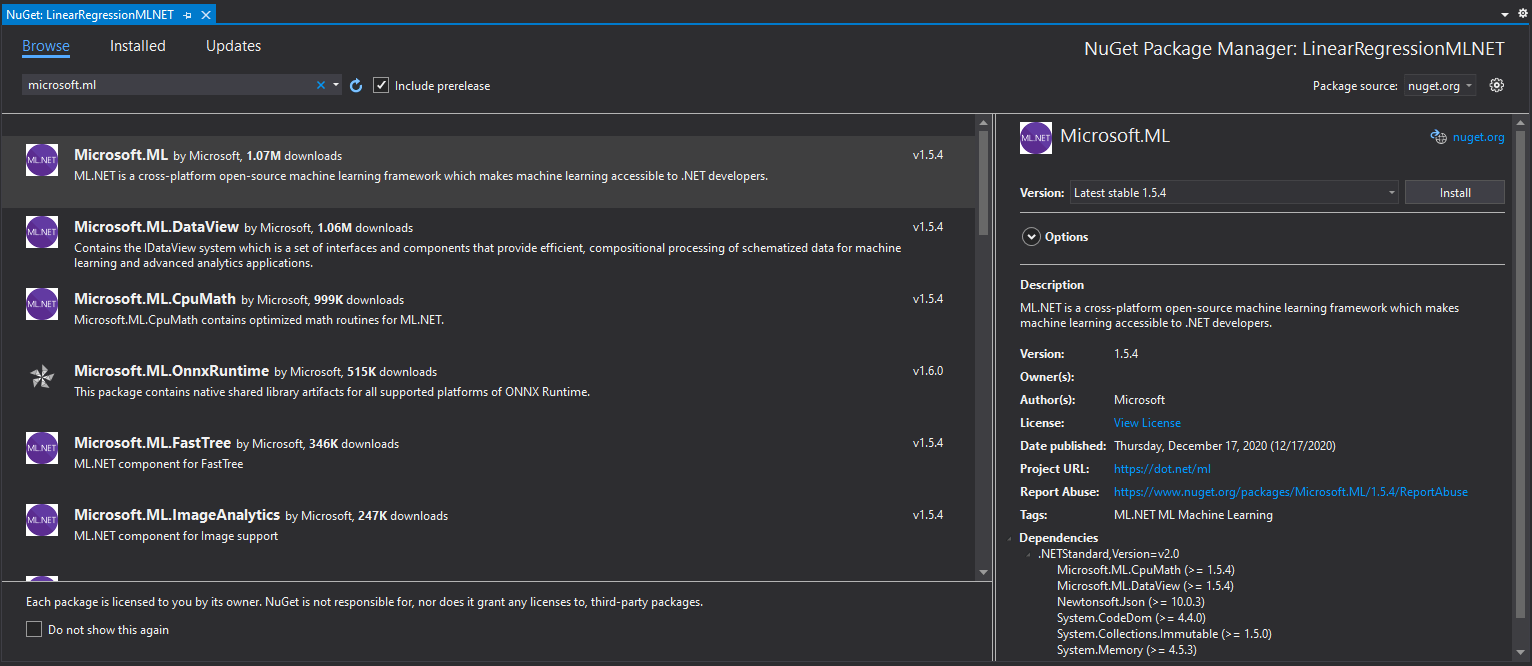If you need to catch up with the basics of machine learning with ML.NET check out this article. Apart from that, you should be comfortable with the basics of linear algebra.

## 2. Simple Linear Regression Theory

Sometimes data that we have is quite simple. Sometimes, the output value of the dataset is just the linear combination of features in the input example. Let’s simplify it even further and say that we have only one feature in the input data. A mathematical model that describes such a relationship can be is presented with the formula:For example, let’s say that this is our data:In this particular case, the mathematical model that we want to create is just a linear function of the input feature, where b0 and b1 are the model’s parameters. These parameters should be learned during the training process. After that, the model should be able to give correct output predictions for new inputs. To sum it up, during training we need to learn b0 and b1 based on the values of x and y, so our f(xi) is able to return correct predictions for the new inputs. If we want to generalize even further we can say that model makes a prediction by adding a constant (bias term – b0) on the precomputed weighted sum (b1) of the input features. However, let’s back to our example and clear things up a little bit before we dive into generalization. Here is what the aforementioned data looks like on the plot:Our linear regression model, by calculating optimal b0 and b1, produces a line that will best fit this data. This line should be optimally distanced from all points in the graph. It is called the regression line. So, how does the algorithm calculates b0 and b1 values?In the formula above, f(xi) represents the predicted output value for ith example from the input, and b0 and b1 are regression coefficients that represent the y-intercept and slope of the regression line. We want that value to be as close as possible to the real value – y. Thus model needs to learn the values regression coefficients b0 and b1, based on which model will be able to predict the correct output. In order to make these estimates, the algorithm needs to know how bad are his current estimations of these coefficients. At the beginning of the training process, we feed samples into the algorithm which calculates output f(xi) of the current sample, based on initial values of regression coefficients. Then the error is calculated and coefficients are corrected. Error for each sample can be calculated like this:Meaning, we subtract estimated output from the real output. Note that this is a training process and we know the value of the output in the i-th sample. Because ei depends on coefficient values it can be described by the function. If we want to minimize ei and for that, we need to define a function based on which we will do so. In this article, we use the Least Squares Technique and define the function that we want to minimize as: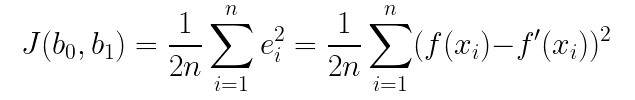The function that we want to minimize is called the objective function or loss function. In order to minimize ei, we need to find coefficients b0 and b1 for which J will hit the global minimum. Without going into mathematical details (you can check out that here), here is how we can calculate values for b0 and b1:Here SSxy is the sum of cross-deviations of y and x: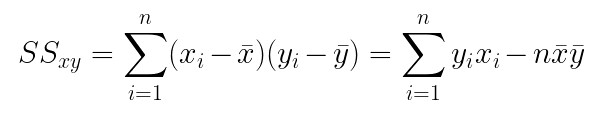while SSxx is the sum of squared deviations of x:Ok, so much for the theory, let’s implement this algorithm using C#.

## 3. Simple Linear Regression C# Implementation

Let’s implement a class that can do simple linear regression with two parameters as we explained in the previous section.

``````using System.Linq;

namespace LinearRegressionFromScratch
{
/// <summary>
/// Simple Linear Regression implementation.
/// Performs linear regression on one feature and one output value.
/// </summary>
public class LinearRegressor
{
private float _b0;
private float _b1;

public LinearRegressor()
{
_b0 = 0;
_b1 = 0;
}

/// <summary>
/// Train Linear Regression algoritm.
/// </summary>
/// <param name="X">Input Data</param>
/// <param name="y">Output Data</param>
public void Fit(float[] X, float[] y)
{
var ssxy = X.Zip(y, (a, b) => a * b).Sum() - X.Length * X.Average() * y.Average();
var ssxx = X.Zip(X, (a, b) => a * b).Sum() - X.Length * X.Average() * X.Average();

_b1 = ssxy / ssxx;
_b0 = y.Average() - _b1 * X.Average();
}

/// <summary>
/// Predict new values.
/// </summary>
/// <param name="x">Input Data</param>
/// <returns>Predictions from the trained algoritm.</returns>
public float[] Predict(float[] x)
{
return x.Select(i => _b0 + i * _b1).ToArray();
}
}
}``````Our LinearRegressor class is quite simple. Following the linear regression formula, this class has two fields _b0 and _b1 which are set to zero in the constructor. Following the usual notation, there are two public methods Fit() and Predict(). The Fit() method is where we perform the training process, while Predict() method creates predictions based on that training process. Here is how we use this class:

``````using System;
using System.Linq;

namespace LinearRegressionFromScratch
{
class Program
{
static void Main(string[] args)
{
float[] X = { 1, 2, 3, 4, 5, 6, 7, 8, 9, 10, 11 };
float[] y = { 6, 6, 11, 17, 16, 20, 23, 23, 29, 33, 39 };

var linearRegressor = new LinearRegressor();
linearRegressor.Fit(X, y);

var predictions = linearRegressor.Predict(X);

Console.WriteLine("Predictions:");
Console.WriteLine(\$"{string.Join(", ", predictions.Select(p => p.ToString()))}");

Console.WriteLine("Actual Value:");
Console.WriteLine(\$"{string.Join(", ", y.Select(p => p.ToString()))}");
}
}
}``````

Here we defined one array for the input values X and an array of the output values y. All we need is to create a LinearRegressor object and train it using Fit() method. Once that is done, we can make predictions. Here we use the same values that we used for training, which is not the best approach and should be avoided, however since this is just an educational example we will give it a pass. Here is the result that we get when we run this code:

``````Predictions:
4.545454, 7.690909, 10.836364, 13.981818, 17.127274, 20.272728, 23.418182, 26.563637, 29.709091,
32.854546, 36
Actual Value:
6, 6, 11, 17, 16, 20, 23, 23, 29, 33, 39``````

We can see that predictions are close, but not quite there. If we visualize those predictions here is what we get: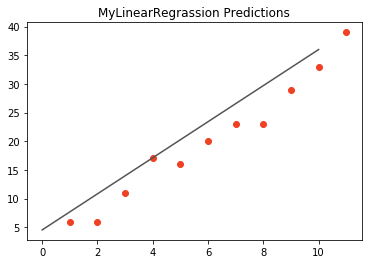Overall it is a nice approximation.

## 4. Multiple Linear Regression Theory

Ok, that was super simple. The usage of this example is very limited since we usually end up with datasets with more features in them. Let’s take it up a notch and get a little more practical…and mathematical. We observe a set of labeled samples {(xi, yi)} Ni=1. The N is the size of the set, while xi is the D-dimensional feature vector and yi is the output. Every feature x is the real number. One such dataset is the famous Boston Housing Dataset. Here is what it looks like: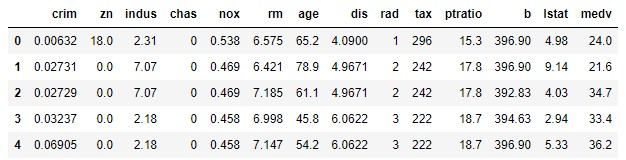In this dataset, the output is the medv feature, while the rest of the features are input features. As you can see there are several features (x1 – crim, x2-zn,….) for each sample i. Now, we can generalize principles of linear regression and use them on a dataset with more features. We can present the model with a formula: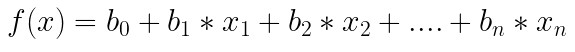Or to simplify it even further: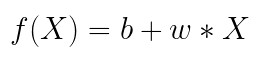We changed the notion there a little bit, but it is essentially the same as the previous formula, it is just vectorized. The bias b0 became b. The w is now a D-dimensional vector (because we have a D number of features, remember) of parameters. To predict the y for a given x we use this model.Obviously, we want to find the optimal values for coefficients (w, b) for which the model will output accurate predictions. Unlike simple linear regression which creates a line, multiple linear regression creates a hyperplane, since every feature represents one dimension. This hyperplane is chosen like that to be as close to all sample values as possible. To calculate the optimal coefficient, this time we want to minimize  Mean Squared Error function: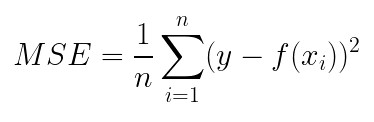To quickly find the values of w and b that minimize MSE we use the so-called Normal Equation. This equation gives direct results for mentioned coeficients:Ok, let’s utilize this in the code.

## 5. Multiple Linear Regression C# Implementation

The algorithm we discussed previously is implemented withing MultipleLinearRegressor class:

``````using MathNet.Numerics.LinearAlgebra;
using System;
using System.Linq;

namespace MultipleLinearRegressionFromScratch
{
/// <summary>
/// Implementation of Multiple Linear Regression.
/// </summary>
public class MultipleLinearRegressor
{
private double _b;
private double[] _w;

public MultipleLinearRegressor()
{
_b = 0;
}

public void Fit(double[,] X, double[,] y)
{
var input = ExtendInputWithOnes(X);
var output = Matrix<double>.Build.DenseOfArray(y);

var coeficients = ((input.Transpose() * input).Inverse() * input.Transpose() * output)
.Transpose().Row(0);
_b = coeficients.ElementAt(0);
_w = SubArray(coeficients.ToArray(), 1, X.GetLength(1));
}

public double Predict(double[,] x)
{
var input = Matrix<double>.Build.DenseOfArray(x).Transpose();
var w = Vector<double>.Build.DenseOfArray(_w);
return input.Multiply(w).ToArray().Sum() + _b;
}

private Matrix<double> ExtendInputWithOnes(double[,] X)
{
// Add 'ones' to the input array to model coefficient b in data.
var ones = Matrix<double>.Build.Dense(X.GetLength(0), 1, 1d);
var extendedX = ones.Append(Matrix<double>.Build.DenseOfArray(X));

return extendedX;
}

private double[] SubArray(double[] data, int index, int length)
{
double[] result = new double[length];
Array.Copy(data, index, result, 0, length);
return result;
}
}
}``````

That is a lot of code so let’s explain it in more detail. This class has two fields _b and _w. They represent parameters of this machine learning algorithm that will be changed during the training process. There are two private methods ExtendInputWithOnes and SubArray. Since we want to learn parameters in one shot and parameter b from the equation is not modeled in the data, we need to extend the input matrix with one column with all ones. This is one in ExtendInputWithOnes method. The SubArray method retrives sub-array from the passed array. Apart from that, we have two public functions Fit() and Predict(), just like in the previous implementation. In the Fit() method we utilize what we have learned from theory and train our algorithm, while we make new predictions with Predict() method. Note the use of the MathNet library.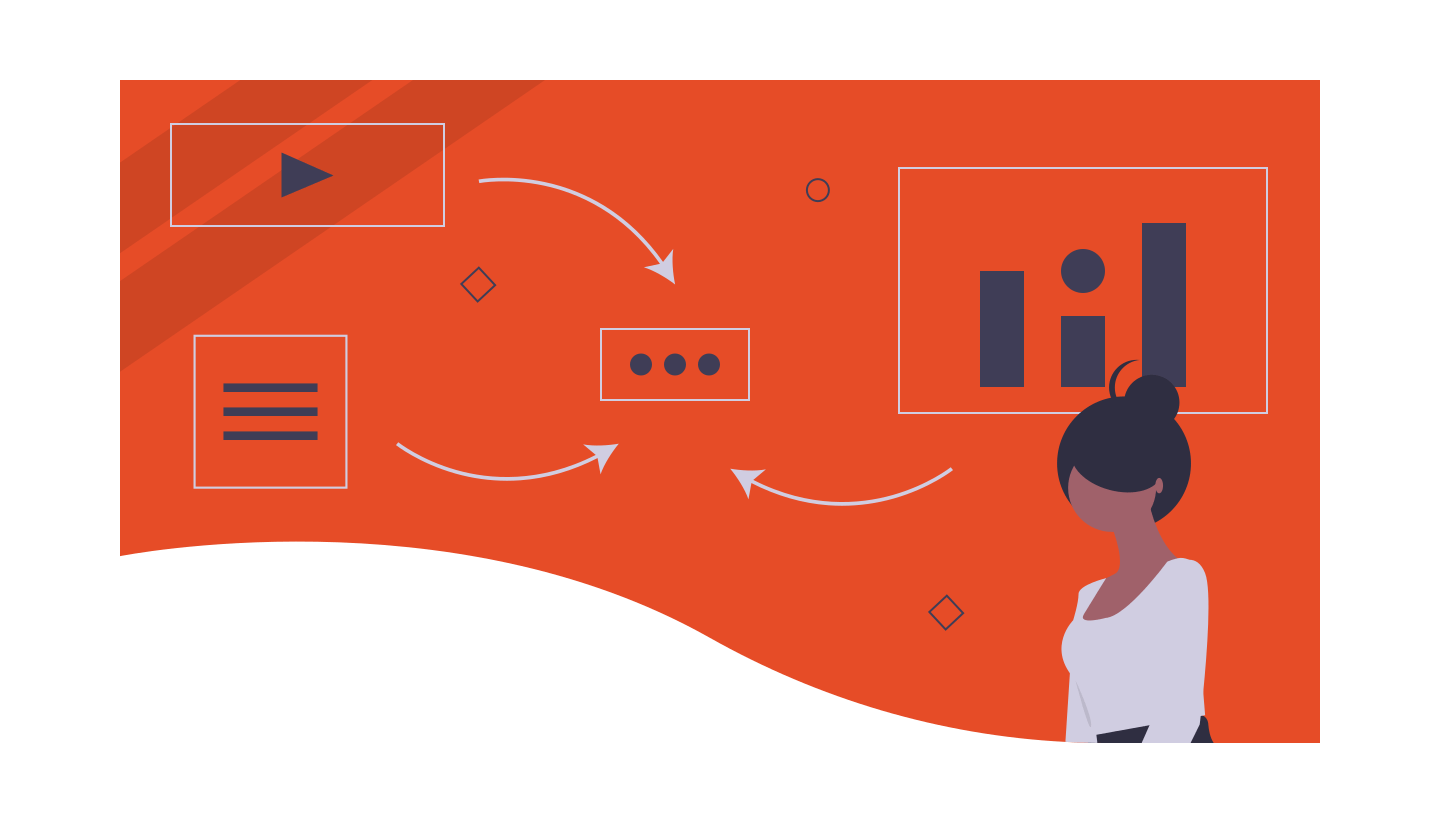Let’s use this class:

``````using System;

namespace MultipleLinearRegressionFromScratch
{
class Program
{
static void Main(string[] args)
{
double[,] X = { { 1, 2, 3},
{ 2, 9, 11},
{ 56, 111, 66}};

double[,] y = { { 6 }, { 6 }, { 11 } };

var linearRegressor = new MultipleLinearRegressor();
linearRegressor.Fit(X, y);

var prediction = linearRegressor.Predict(new double[,] { { 3 } , { 5 } , { 7 } });

Console.WriteLine(\$"Prediction: {prediction}");
}
}
}``````

We use some dummy data just to demonstrate how this class functions. In the end for the new sample we get this prediction:

``Prediction: 93.6015625``

## 6. ML.NET Linear Regression Algorithms

In ML.NET we don’t have these plain implementations of the Linear Regression, but we have some which are more advanced. There are two improved variations of Linear Regression that you can use with ML.NET:

• Online Gradient DescentStochastic gradient descent is one of the most popular machine learning algorithms. It uses a simple yet efficient iterative technique to fit model coefficients using error gradients. With these iterations, it avoids memory problems, which we might face if we try to load a large dataset in our vanilla implementations. Online Gradient Descent is a variation of the Stochastic Gradient descent with a choice of loss functions, and an option to update the weight vector using the average of the vectors seen over time.
• SDCAStochastic Dual Coordinate Ascent (SDCA) is another variation on Stochastic Gradient Descent which is suitable for large dataset. The algorithm can be scaled because it’s a streaming training algorithm. This algorithm a state-of-the-art optimization technique for convex objective functions. You can find out more about it in this paper.

In the next section, we use these algorithms on Boston Housing Dataset.

## 7. ML.NET Implementation

Before we dive into the ML.NET implementation, let’s consider the high-level architecture of this implementation. In general, we want to build an easily extendable solution that we can easily extend with new linear algorithms that ML.NET might include in the future. That is why the folder structure of our solution looks like this:The Data folder contains .csv with input data and the MachineLearning folder contains everything that is necessary for our algorithm to work. The architectural overview can be represented like this: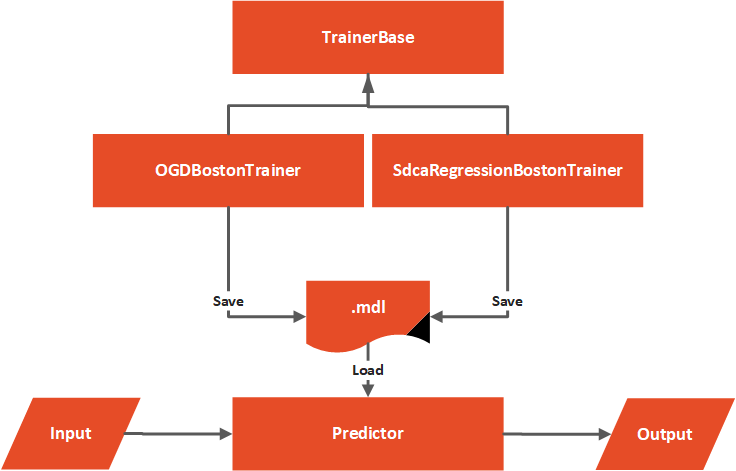At the core of this solution, we have an abstract TrainerBase class. This class is in the Common folder and its main goal is to standardize the way this whole process is done. It is in this class where we train our machine learning algorithm. The classes that implement this abstract class are located in the Trainers folder. Here we can find two classes OGDBostonTrainer and SdcaRegressionBostonTrainer. These classes define which algorithm should be used and how the data should be pre-processed. In this particular case, we have only one Predictor located in the Predictor folder.

### 7.1 Data Models

In order to load data from the dataset and use it with ML.NET algorithms, we need to implement classes that are going to model this data. Two files can be found in Data Folder: BostonHousingData and BostonHousingPricePredictions. The BostonHousingData class models input data and it looks like this:

``````using Microsoft.ML.Data;

namespace LinearRegressionMLNET.MachineLearning.DataModels
{
/// <summary>
/// Models Boston Housing Data.
/// </summary>
public class BostonHousingData
{
public float CrimeRate { get; set; }

public float Zoned { get; set; }

public float Proportion { get; set; }

public float RiverCoast { get; set; }

public float NOConcetration { get; set; }

public float NumOfRoomsPerDwelling { get; set; }

public float Age { get; set; }

public float EmployCenterDistance { get; set; }

public float HighwayAccecabilityRadius { get; set; }

public float TaxRate { get; set; }

public float PTRatio { get; set; }

public float MedianPrice { get; set; }

}
}``````The BostonHousingPricePredictions class models output data:

``````using Microsoft.ML.Data;

namespace LinearRegressionMLNET.MachineLearning.DataModels
{
/// <summary>
/// Models Boston Housing Prediction.
/// </summary>
public class BostonHousingPricePredictions
{
[ColumnName("Score")]
public float MedianPrice;
}
}``````

### 7.2 TrainerBase Class

As we mentioned, this class is the core of this implementation. Here is what it looks like:

``````using LinearRegressionMLNET.MachineLearning.DataModels;
using Microsoft.ML;
using Microsoft.ML.Data;
using Microsoft.ML.Trainers;
using Microsoft.ML.Transforms;
using System;
using System.IO;

namespace LinearRegressionMLNET.MachineLearning.Common
{
/// <summary>
/// Base class for Trainers.
/// This class exposes methods for training, evaluating and saving ML Models.
/// </summary>
public abstract class TrainerBase
{
public string Name { get; protected set; }

protected static string ModelPath =>
Path.Combine(AppContext.BaseDirectory, "regression.mdl");

protected DataOperationsCatalog.TrainTestData _dataSplit;
protected ITrainerEstimator<RegressionPredictionTransformer
<LinearRegressionModelParameters>, LinearRegressionModelParameters> _model;
protected ITransformer _trainedModel;

protected TrainerBase()
{
MlContext = new MLContext(111);
}

/// <summary>
/// Train model on defined data.
/// </summary>
/// <param name="trainingFileName"></param>
public void Fit(string trainingFileName)
{
if (!File.Exists(trainingFileName))
{
throw new FileNotFoundException(\$"File {trainingFileName} doesn't exist.");
}

var dataProcessPipeline = BuildDataProcessingPipeline();
var trainingPipeline = dataProcessPipeline.Append(_model);

_trainedModel = trainingPipeline.Fit(_dataSplit.TrainSet);
}

/// <summary>
/// Evaluate trained model.
/// </summary>
public RegressionMetrics Evaluate()
{
var testSetTransform = _trainedModel.Transform(_dataSplit.TestSet);

return MlContext.Regression.Evaluate(testSetTransform);
}

/// <summary>
/// Save Model in the file.
/// </summary>
public void Save()
{
MlContext.Model.Save(_trainedModel, _dataSplit.TrainSet.Schema, ModelPath);
}

/// <summary>
/// Feature engeneering and data pre-processing.
/// </summary>
/// <returns>Data Processing Pipeline.</returns>
protected abstract EstimatorChain<NormalizingTransformer> BuildDataProcessingPipeline();

{
return MlContext.Data.TrainTestSplit(trainingDataView, testFraction: 0.3);
}
}
}``````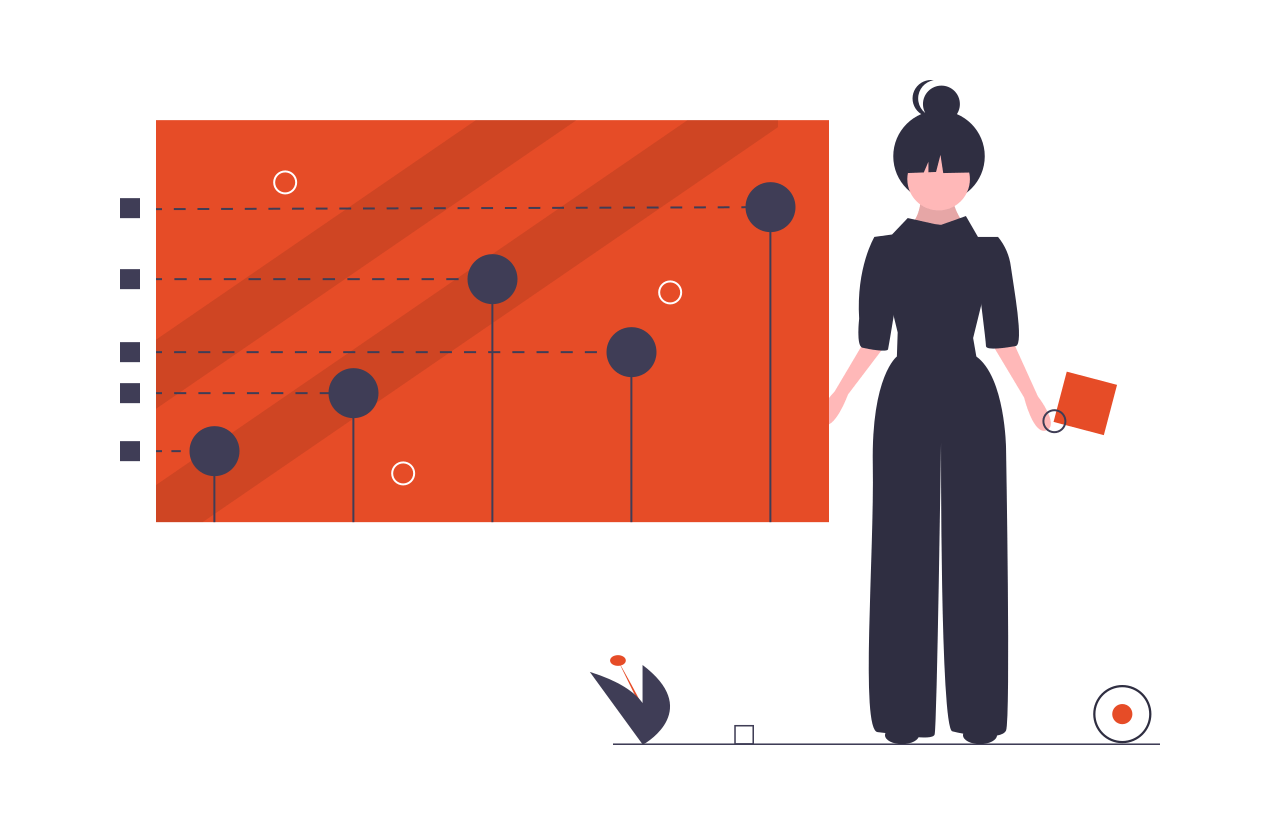That is one large class. It controls the whole process. Let’s split it up and see what it is all about. First, let’s observe the fields and properties of this class:

``````public string Name { get; protected set; }

protected static string ModelPath => Path.Combine(AppContext.BaseDirectory, "regression.mdl");

protected DataOperationsCatalog.TrainTestData _dataSplit;
protected ITrainerEstimator<RegressionPredictionTransformer<LinearRegressionModelParameters>,
LinearRegressionModelParameters> _model;
protected ITransformer _trainedModel;``````

The Name property is used by the class that inherits this one to add the name of the algorithm. The ModelPath field is there to define where we will store our model once it is trained. Note that the file name has .mdl extension. Then we have our MlContext so we can use ML.NET functionalities. Don’t forget that this class is a singleton, so there will be only one in our solution. The _dataSplit field contains loaded data. Data is split into train and test datasets within this structure. The field _model is used by the child classes. These classes define which machine learning algorithm is used in this field. The _trainedModel field is the resulting model that should be evaluated and saved. Cool, let’s now explore Fit() method:

``````public void Fit(string trainingFileName)
{
if (!File.Exists(trainingFileName))
{
throw new FileNotFoundException(\$"File {trainingFileName} doesn't exist.");
}

var dataProcessPipeline = BuildDataProcessingPipeline();
var trainingPipeline = dataProcessPipeline.Append(_model);

_trainedModel = trainingPipeline.Fit(_dataSplit.TrainSet);
}``````

This method is the blueprint for the training of the algorithms. As an input parameter, it receives the path to the .csv file. After we confirm that the file exists we use the private method LoadAndPrepareData. This method loads data into memory and splits it into two datasets, train and test dataset. We store the returning value into _dataSplit because we need a test dataset for the evaluation phase. Then we call BuildDataProcessingPipeline().This is an abstract method that needs to be overridden by the child class. In essence, the only job of the class that inherits and implements this one is to define the algorithm that should be used, by instantiating an object of the desired algorithm as _model and overriding BuildDataProcessingPipeline() so data is correctly prepared for the defined algorithm. We complete the training pipeline by appending _model to the prepared data process pipeline. Finally, we can call the Fit() method of the training pipeline and store the trained model in the _trainedModel field. Next is the Evaluate() method:

``````public RegressionMetrics Evaluate()
{
var testSetTransform = _trainedModel.Transform(_dataSplit.TestSet);

return MlContext.Regression.Evaluate(testSetTransform);
}``````

It is a pretty simple method that creates a Transformer object by using _trainedModel and test Dataset. Then we utilize MlContext to retrieve regression metrics. Finally, let’s check out Save() method:

``````public void Save()
{
MlContext.Model.Save(_trainedModel, _dataSplit.TrainSet.Schema, ModelPath);
}``````

This is another simple method that just uses MLContext to save the model into the defined path.

### 7.3 Trainers

Thanks to all the heavy lifting that we have done in the TrainerBase class, the other Trainer classes are pretty simple and focused only on preparing data for the concrete algorithm. We have two classes that utilize ML.NET‘s Online Gradient Descent and SDCA implementations. First, let’s check out the class OGDBostonTrainer class that implements Online Gradient Descent for Boston housing dataset:

``````using LinearRegressionMLNET.MachineLearning.Common;
using LinearRegressionMLNET.MachineLearning.DataModels;
using Microsoft.ML;
using Microsoft.ML.Data;
using Microsoft.ML.Transforms;

namespace LinearRegressionMLNET.MachineLearning.Trainers
{
/// <summary>
/// Class that uses Online Gradient Descent algorithm.
/// </summary>
public sealed class OGDBostonTrainer : TrainerBase
{
public OGDBostonTrainer() : base()
{
_model = MlContext.Regression.Trainers
}

protected override EstimatorChain<NormalizingTransformer> BuildDataProcessingPipeline()
{
var dataProcessPipeline = MlContext.Transforms
.CopyColumns("Label", nameof(BostonHousingData.MedianPrice))
.Append(MlContext.Transforms.Categorical.OneHotEncoding("RiverCoast"))
.Append(MlContext.Transforms.Concatenate("Features",
"CrimeRate",
"Zoned",
"Proportion",
"RiverCoast",
"NOConcetration",
"NumOfRoomsPerDwelling",
"Age",
"EmployCenterDistance",
"TaxRate",
"PTRatio"))
.Append(MlContext.Transforms.NormalizeLogMeanVariance("Features", "Features"))
.AppendCacheCheckpoint(MlContext);

return dataProcessPipeline;
}
}
}``````In the constructor of this class, we define the name and instantiate ML.NET object of OnlineGradientDescent class. In the overridden BuildDataProcessingPipeline we have done some Data pre-processing and feature engineering. Namely, we used one-hot encoding on the RiverCoast feature and used log mean normalization on all features. The SdcaRegressionBostonTrainer is similar:

``````using LinearRegressionMLNET.MachineLearning.Common;
using LinearRegressionMLNET.MachineLearning.DataModels;
using Microsoft.ML;
using Microsoft.ML.Data;
using Microsoft.ML.Transforms;

namespace LinearRegressionMLNET.MachineLearning.Trainers
{
/// <summary>
/// Class that uses SDCA Regression algorithm.
/// </summary>
public class SdcaRegressionBostonTrainer: TrainerBase
{
public SdcaRegressionBostonTrainer() : base()
{
Name = "SDCA";
_model = MlContext.Regression.Trainers
.Sdca(labelColumnName: "Label", featureColumnName: "Features");
}

protected override EstimatorChain<NormalizingTransformer> BuildDataProcessingPipeline()
{
var dataProcessPipeline = MlContext.Transforms
.CopyColumns("Label", nameof(BostonHousingData.MedianPrice))
.Append(MlContext.Transforms.Concatenate("Features",
"CrimeRate",
"Zoned",
"Proportion",
"RiverCoast",
"NOConcetration",
"NumOfRoomsPerDwelling",
"Age",
"EmployCenterDistance",
"TaxRate",
"PTRatio"))
.Append(MlContext.Transforms.NormalizeLogMeanVariance("Features", "Features"))
.AppendCacheCheckpoint(MlContext);

return dataProcessPipeline;
}
}
}``````

Basically, the only difference is that the _model field is now assigned Sdca class instance.

### 7.4 Predictor

The Predictor class is here to load the saved model and run some predictions. Usually, this class is not a part of the same microservice as trainers. We usually have one microservice that is performing the training of the model. This model is saved into file, from which the other model loads it and run predictions based on the user input. Here is how this class looks like:

``````using LinearRegressionMLNET.MachineLearning.DataModels;
using Microsoft.ML;
using System;
using System.Collections.Generic;
using System.IO;
using System.Text;

namespace LinearRegressionMLNET.MachineLearning.Predictors
{
/// <summary>
/// Loads Model from the file and makes predictions.
/// </summary>
public class Predictor
{
protected static string ModelPath =>
Path.Combine(AppContext.BaseDirectory, "regression.mdl");

private ITransformer _model;

public Predictor()
{
_mlContext = new MLContext(111);
}

/// <summary>
/// Runs prediction on new data.
/// </summary>
/// <param name="newSample">New data sample.</param>
public BostonHousingPricePredictions Predict(BostonHousingData newSample)
{

var predictionEngine = _mlContext.Model
.CreatePredictionEngine<BostonHousingData, BostonHousingPricePredictions>(_model);

return predictionEngine.Predict(newSample);
}

{
if (!File.Exists(ModelPath))
{
throw new FileNotFoundException(\$"File {ModelPath} doesn't exist.");
}

using (var stream = new FileStream(
ModelPath,
FileMode.Open,
{
}

if (_model == null)
{
throw new Exception(\$"Failed to load Model");
}
}
}
}``````

In a nutshell, the model is loaded from a defined file, and predictions are made on the new sample. Note that we need to create PredictionEngine to do so.### 7.4 Usage and Results

Ok, let’s put all of this together.

``````using LinearRegressionMLNET.MachineLearning.Common;
using LinearRegressionMLNET.MachineLearning.DataModels;
using LinearRegressionMLNET.MachineLearning.Predictors;
using LinearRegressionMLNET.MachineLearning.Trainers;
using System;

namespace LinearRegressionMLNET
{
class Program
{
static void Main(string[] args)
{
var newSample = new BostonHousingData
{
Age = 65.2f,
CrimeRate = 0.00632f,
EmployCenterDistance = 4.0900f,
NOConcetration = 0.538f,
NumOfRoomsPerDwelling = 6.575f,
Proportion = 1f,
PTRatio = 15.3f,
RiverCoast = 0f,
TaxRate = 296f,
Zoned = 23f
};

var ogd = new OGDBostonTrainer();
TrainEvaluatePredict(ogd, newSample);

var sdca = new SdcaRegressionBostonTrainer();
TrainEvaluatePredict(sdca, newSample);
}

static void TrainEvaluatePredict(TrainerBase trainer, BostonHousingData newSample)
{
Console.WriteLine("*******************************");
Console.WriteLine(\$"{ trainer.Name }");
Console.WriteLine("*******************************");

trainer.Fit("..\\Data\\boston_housing_dataset.csv");

var modelMetrics = trainer.Evaluate();

Console.WriteLine(\$"Loss Function: {modelMetrics.LossFunction:0.##}{Environment.NewLine}" +
\$"Mean Absolute Error: {modelMetrics.MeanAbsoluteError:#.##}{Environment.NewLine}" +
\$"Mean Squared Error: {modelMetrics.MeanSquaredError:#.##}{Environment.NewLine}" +
\$"RSquared: {modelMetrics.RSquared:0.##}{Environment.NewLine}" +
\$"Root Mean Squared Error: {modelMetrics.RootMeanSquaredError:#.##}");

trainer.Save();

var predictor = new Predictor();
var prediction = predictor.Predict(newSample);
Console.WriteLine("------------------------------");
Console.WriteLine(\$"Prediction: {prediction.MedianPrice:#.##}");
Console.WriteLine("------------------------------");
}
}
}``````

Not the TrainEvaluatePredict() method. This method does the heavy lifting here. In this method, we can inject an instance of the class that inherits TrainerBase and a new sample that we want to be predicted. Then we call Fit() method to train the algorithm. Then we call Evaluate() method and print out the metrics. Finally, we save the model. Once that is done, we create an instance of Predictor, call Predict() method with a new sample and print out the predictions. In the Main, we create an instance of OGDBostonTrainer class and run TrainEvaluatePredict with it. Then we do the same for SdcaRegressionBostonTrainer. Here are the results:

``````*******************************
*******************************
Loss Function: 8.25
Mean Absolute Error: 2.49
Mean Squared Error: 8.25
RSquared: -0.67
Root Mean Squared Error: 2.87
------------------------------
Prediction: 20.38
------------------------------
*******************************
SDCA
*******************************
Loss Function: 3.05
Mean Absolute Error: 1.33
Mean Squared Error: 3.05
RSquared: 0.39
Root Mean Squared Error: 1.75
------------------------------
Prediction: 16.13
------------------------------``````

Observing the metrics we can say that SDCA performed better and consider this estimation to be a better one.

## Conclusion

In this article, we covered a lot of things. First, we explored Linear regression and Multiple Linear Regression in-depth and then we implement both of those algorithms from scratch. Then we used ML.NET‘s algorithms like Online Gradient Descent and SDCA. We created a robust and extendable solution for ML.NET and run the predictions on Boston Housing Dataset. Pretty neat. What do you think?#### Nikola M. Zivkovic

CAIO at Rubik's Code

Nikola M. Zivkovic a CAIO at Rubik’s Code and the author of book “Deep Learning for Programmers“. He is loves knowledge sharing, and he is experienced speaker. You can find him speaking at meetups, conferences and as a guest lecturer at the University of Novi Sad.

Rubik’s Code is a boutique data science and software service company with more than 10 years of experience in Machine Learning, Artificial Intelligence & Software development. Check out the services we provide.

Ultimate Guide to Machine Learning with Python

Everything from Python basics to the deployment of Machine Learning algorithms to production in one place.

Become a Machine Learning Superhero TODAY!